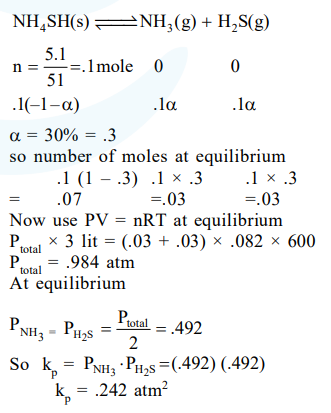# 5.1g NH4SH is introduced in 3.0 L

Question:

$5.1 \mathrm{~g} \mathrm{NH} \mathrm{NH}_{4} \mathrm{SH}$ is introduced in $3.0 \mathrm{~L}$ evacuated flask at $327^{\circ} \mathrm{C} .30 \%$ of the solid $\mathrm{NH}_{4} \mathrm{SH}$ decomposed to $\mathrm{NH}_{3}$ and $\mathrm{H}_{2} \mathrm{~S}$ as gases. The $\mathrm{K}_{\mathrm{p}}$ of the reaction at $327^{\circ} \mathrm{C}$ is $(\mathrm{R}=0.082 \mathrm{~L}$ atm $\mathrm{mol}^{-1} \mathrm{~K}^{-1}$, Molar mass of $\mathrm{S}=32 \mathrm{~g} \mathrm{~mol}^{01}$, molar mass of $\mathrm{N}=14 \mathrm{~g} \mathrm{~mol}^{-1}$ )

1. $1 \times 10^{-4} \mathrm{~atm}^{2}$

2. $4.9 \times 10^{-3} \mathrm{~atm}^{2}$

3. $0.242 \mathrm{~atm}^{2}$

4. $0.242 \times 10^{-4} \mathrm{~atm}^{2}$

Correct Option: , 3

Solution: